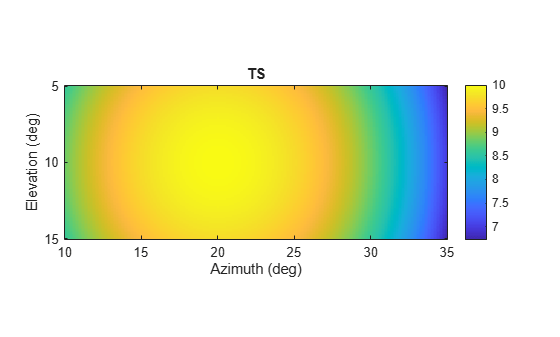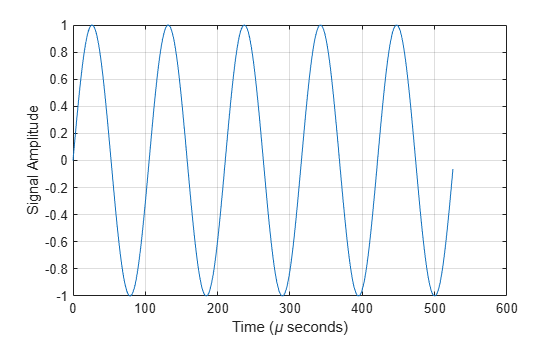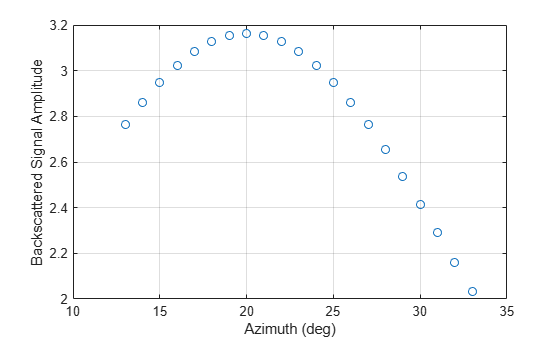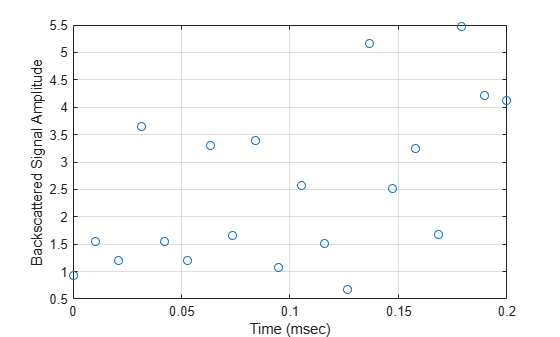# step

System object: phased.BackscatterSonarTarget
Package: phased

Backscatter incoming sonar signal

## Syntax

refl_sig = step(target,sig,ang)
refl_sig = step(target,sig,ang,update)

## Description

Note

Instead of using the step method to perform the operation defined by the System object™, you can call the object with arguments, as if it were a function. For example, y = step(obj,x) and y = obj(x) perform equivalent operations.

example

refl_sig = step(target,sig,ang) returns the reflected signal, refl_sig, of an incident sonar signal, sig, arriving at the target from the angle, ang.

example

refl_sig = step(target,sig,ang,update) uses update to control whether to update the target strength (TS) values. This syntax applies when you set the Model property to one of the fluctuating TS models: 'Swerling1', 'Swerling2', 'Swerling3', or 'Swerling4'. If update is true, a new TS value is generated. If update is false, the previous TS value is used.

Note

The object performs an initialization the first time the object is executed. This initialization locks nontunable properties and input specifications, such as dimensions, complexity, and data type of the input data. If you change a nontunable property or an input specification, the System object issues an error. To change nontunable properties or inputs, you must first call the release method to unlock the object.

## Input Arguments

expand all

Backscatter sonar target, specified as a phased.BackscatterSonarTarget System object.

Sonar signal, specified as an N-by-M complex-valued matrix. The quantity N is the number of signal samples and M is the number of signals reflecting off the target. Each column corresponds to an independent signal incident at a different reflecting angle.

When you specify the TSPattern property as a Q-by-P-by-M, a separate pattern is used for each signal. When you specify TSPattern as a Q-by-Pmatrix, the same pattern is used for every signal.

The size of the first dimension of the input matrix can vary to simulate a changing signal length. A size change can occur, for example, in the case of a pulse waveform with variable pulse repetition frequency.

Example: [1,1;j,1;0.5,0]

Data Types: double
Complex Number Support: Yes

Incident signal direction, specified as a 2-by-1 positive real-valued column vector or a 2-by-M positive real-valued column matrix. Each column of ang specifies the incident direction of the corresponding signal in the form of an [AzimuthAngle;ElevationAngle] pair. Units are degrees. The number of columns in ang must match the number of independent signals in sig.

Example: [30;45]

Data Types: double

Allow the TS values for fluctuation models to update, specified as false or true. When update is true, a new TS value is generated with each call to the step method. If update is false, TS remains unchanged with each call to step.

Example: true

Data Types: logical

## Output Arguments

expand all

Narrowband reflected sonar signal, specified as an N-by-M complex-valued matrix. Each column contains an independent signal reflected from the target.

The quantity N is the number of signal samples and M is the number of signals reflecting off the target. Each column corresponds to a reflecting angle.

The output refl_sig contains signal samples arriving at the signal destination within the current input time frame. When the propagation time from source to destination exceeds the current time frame duration, the output will not contain all contributions from the input of the current time frame. The remaining output appears in the next call to step.

## Examples

expand all

Calculate the reflected sonar signal from a nonfluctuating point target with a peak target strength (TS) of 10.0 db. For illustrative purposes, use a simplified expression for the TS pattern of a target. Real TS patterns are more complicated. The TS pattern covers a range of angles from 10° to 30° in azimuth and from 5° to 15° in elevation. The TS peaks at 20° azimuth and 10° elevation. Assume that the sonar operating frequency is 10 kHz and that the signal is a sinusoid at 9500 kHz.

Create and plot the TS pattern.

azmax = 20.0; elmax = 10.0; azpatangs = [10.0:0.1:35.0]; elpatangs = [5.0:0.1:15.0]; tspattern = 10.0*cosd(4*(elpatangs - elmax))'*cosd(4*(azpatangs - azmax)); tspatterndb = 10*log10(tspattern); imagesc(azpatangs,elpatangs,tspatterndb) colorbar axis image axis tight title('TS') xlabel('Azimuth (deg)') ylabel('Elevation (deg)')Generate and plot 50 samples of the sonar signal.

freq = 9.5e3; fs = 100*freq; nsamp = 500; t = [0:(nsamp-1)]'/fs; sig = sin(2*pi*freq*t); plot(t*1e6,sig) xlabel('Time (\mu seconds)') ylabel('Signal Amplitude') gridCreate the phased.BackscatterSonarTarget System object™.

target = phased.BackscatterSonarTarget('Model','Nonfluctuating', ... 'AzimuthAngles',azpatangs,'ElevationAngles',elpatangs, ... 'TSPattern',tspattern);

For a sequence of different azimuth incident angles (at constant elevation angle), plot the maximum scattered signal amplitude.

az0 = 13.0; el = 10.0; naz = 20; az = az0 + [0:1:20]; naz = length(az); ss = zeros(1,naz); for k = 1:naz y = target(sig,[az(k);el]); ss(k) = max(abs(y)); end plot(az,ss,'o') xlabel('Azimuth (deg)') ylabel('Backscattered Signal Amplitude') gridCalculate the reflected sonar signal from a Swerling2 fluctuating point target with a peak target strength (TS) of 10.0 db. For illustrative purposes, use a simplified expression for the TS pattern of a target. Real TS patterns are more complicated. The TS pattern covers a range of angles from 10°to 30° in azimuth and from 5° ro 15° in elevation. The TS peaks at 20° azimuth and 10° elevation. Assume that the sonar operating frequency is 10 kHz and that the signal is a sinusoid at 9500 kHz.

Create and plot the TS pattern.

azmax = 20.0; elmax = 10.0; azpatangs = [10.0:0.1:35.0]; elpatangs = [5.0:0.1:15.0]; tspattern = 10.0*cosd(4*(elpatangs - elmax))'*cosd(4*(azpatangs - azmax)); tspatterndb = 10*log10(tspattern); imagesc(azpatangs,elpatangs,tspatterndb) colorbar axis image axis tight title('TS') xlabel('Azimuth (deg)') ylabel('Elevation (deg)')Generate the sonar signal.

freq = 9.5e3; fs = 10*freq; nsamp = 50; t = [0:(nsamp-1)]'/fs; sig = sin(2*pi*freq*t);

Create the phased.BackscatterSonarTarget System object™.

target = phased.BackscatterSonarTarget('Model','Nonfluctuating',... 'AzimuthAngles',azpatangs,'ElevationAngles',elpatangs,... 'TSPattern',tspattern,'Model','Swerling2');

Compute and plot the fluctuating signal amplitude for 20 time steps.

az = 20.0; el = 10.0; nsteps = 20; ss = zeros(1,nsteps); for k = 1:nsteps y = target(sig,[az;el],true); ss(k) = max(abs(y)); end plot([0:(nsteps-1)]*1000/fs,ss,'o') xlabel('Time (msec)') ylabel('Backscattered Signal Amplitude') grid## Version History

Introduced in R2017a